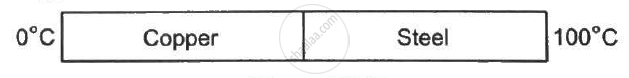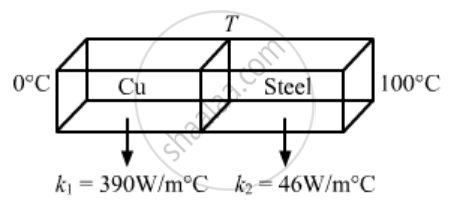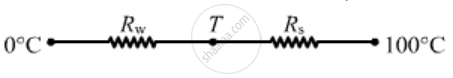Share

# Figure (28-E2) shows a copper rod joined to a steel rod. The rods have equal length and equal cross sectional area. The free end of the copper rod is kept at 0°C and that of the steel rod is kept at - Physics

ConceptThermal Expansion of Solids

#### Question

Figure (28-E2) shows a copper rod joined to a steel rod. The rods have equal length and equal cross sectional area. The free end of the copper rod is kept at 0°C and that of the steel rod is kept at 100°C. Find the temperature at the junction of the rods. Conductivity of copper = 390 W m−1°C−1 and that if steel = 46 W m−1°C−1.#### SolutionRods are connected in series, so the rate of flow of heat is same.Rate of flow of heat is given by

q = (dQ)/(dt) = "Temperature differences"/ "Thermal resistance"

As q1 = q2
⇒ T-0 / (R_{cu}) =(100 - T ) /(R_s)

Ak_1 ((T - 0)/(l)) = (100 - T ) 46

⇒ 390 T = (100 - T) 46

⇒ T = 10.6° C

Is there an error in this question or solution?

#### APPEARS IN

Solution Figure (28-E2) shows a copper rod joined to a steel rod. The rods have equal length and equal cross sectional area. The free end of the copper rod is kept at 0°C and that of the steel rod is kept at Concept: Thermal Expansion of Solids.
S